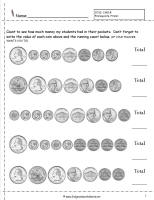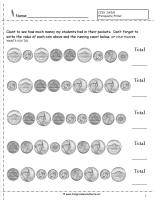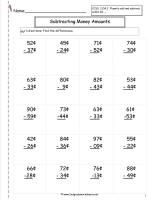﻿ Counting Coins and Money Worksheets and Printouts

Coin Values, Counting Coins, Counting Money

Coin Values, Counting Coins, Determine Value of Coins, Counting MoneyCoin Identification and Values

Common Core State Standards: 2.MD.8  Work with time and money.
Reference page for coin identification and value.Counting Coins Introduction

Common Core State Standards: 2.MD.8  Work with time and money.
Page explaining how to count coin values to determine money amount.Counting Coins Worksheets Without Quarters

Common Core State Standards: 2.MD.8  Work with time and money.
Students count coin values to determine amount of money.  Coins are in order by value.  There are no quarters on these worksheets.  No half dollars.  3 pages.Counting Coins Worksheets With Quarters

Common Core State Standards: 2.MD.8  Work with time and money.
Students count coin values to determine amount of money.  Coins are in order by value.  There are quarters on these worksheets.  No half dollars. 3 pages.Counting Coins Worksheets Without Quarters

Common Core State Standards: 2.MD.8  Work with time and money.
Students count coin values to determine amount of money.  Coins are NOT in order by value.  There are no quarters on these worksheets.  No half dollars. 3 pages.Counting Coins Worksheets With Quarters

Common Core State Standards: 2.MD.8  Work with time and money.
Students count coin values to determine amount of money.  Coins are NOT in order by value.  There are quarters on these worksheets.  No half dollars. 3 pages.Counting Coins Skip Counting Practice Worksheet

Common Core State Standards: 2.MD.8  Work with time and money.
For RTI or struggling students.  Practice skip counting and then apply it to counting coins.  5 pages.Counting Coins Skip Counting With Hundred Chart

Common Core State Standards: 2.MD.8  Work with time and money.
For RTI or struggling students.  Practice skip counting and then apply it to counting coins.  Includes a hundred chart. 3 pages.Adding Two Digit Money Amounts

Common Core State Standards:  2.MD.8  Work with time and money.   2.NBT.5 Fluently add and subtract within 100....
Students add two-digit money amounts.  Some regrouping is required. 3 pages.Subtracting Two Digit Money Amounts

Common Core State Standards:  2.MD.8  Work with time and money.   2.NBT.5 Fluently add and subtract within 100....
Students subtract two-digit money amounts.  Some regrouping is required. 3 pages.Adding and Subtracting Two Digit Money Amounts

Common Core State Standards:  2.MD.8  Work with time and money.   2.NBT.5 Fluently add and subtract within 100....
Students subtract two-digit money amounts.  Some regrouping is required. 3 pages.